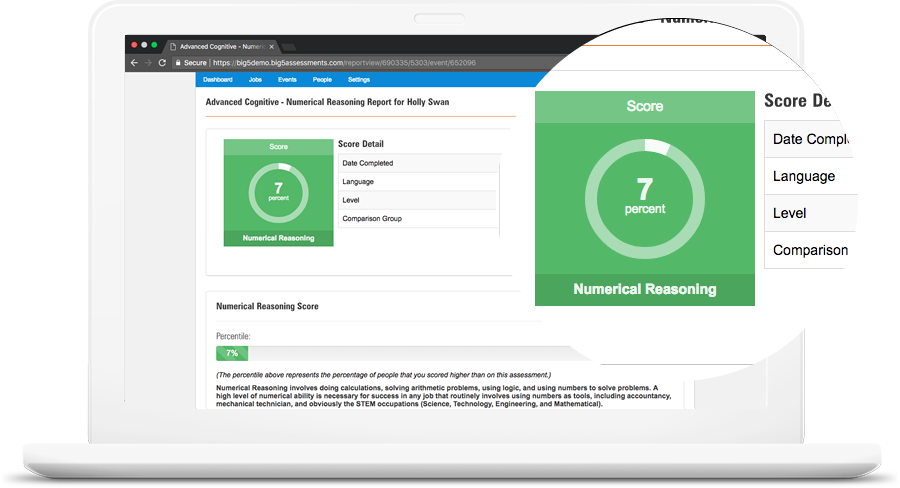# Numerical Reasoning Test

The Big 5 Numerical Reasoning Test measures an individuals numerical reasoning ability.

## Numerical Reasoning Test

Essential...if the candidate needs to be good with numbers and figures for success in the role.

Numerical Reasoning involves doing calculations, solving arithmetic problems, using logic, and using numbers to solve problems. A high level of numerical ability is necessary for success in any job that routinely involves using numbers as tools, including accountancy, mechanical technician, and obviously the STEM occupations (Science, Technology, Engineering, and Mathematical).### Test Summary

 Test Advanced Numerical Reasoning Test A participant’s numerical reasoning ability. The ability to quickly and accurately solve number related problems. A 25 item test that measures numerical reasoning 10-minutes (timed test) View Pricing Plans